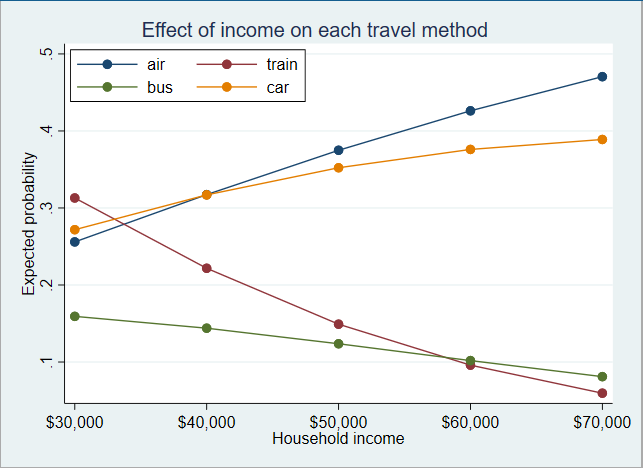Discrete-choice data

Alternative-specific and case-specific covariates

Balanced and unbalanced choice sets

One selected outcome per case or ranked outcomes

Conditional logit models

Odds ratios

Robust, cluster–robust, bootstrap, and jackknife standard errors

Mixed logit models

Also known as

Mixed multinomial logit models

Mixed discrete choice models

Discrete choice models with random coefficients

Random-effect and random-coefficient distributions

Normal

Correlated normal

Lognormal

Truncated normal

Uniform

Triangular

Cross-sectional or panel data

Relaxes IIA assumption

Robust, cluster–robust, bootstrap, and jackknife standard errors

Survey data support

Multinomial probit models

Homo- or heteroskedastic variances

Various correlation structures, including user-specified

Relaxes IIA assumption

Probabilities based on GHK simulator

Robust, cluster–robust, bootstrap, and jackknife standard errors

Nested logit models

Random-utilities maximization model

Full maximum-likelihood estimation

Up to eight nested levels

Facilities to set up the data and display the tree structure

Predictions for utility functions, probabilities, conditional probabilities, and inclusive values

Robust, cluster–robust, bootstrap, and jackknife standard errors

Linear constraints, including constraints on inclusive-value parameters

Summarize choice data

Tabulate choice sets

Summarize covariates by alternative

Tabulate covariates by chosen alternative

Report potential problems in data

Rank-ordered probit models

Plackett–Luce model, exploded logit, choice-based conjoint analysis

Homo- or heteroskedastic variances

Various correlation structures, including user-specified

Relaxes IIA assumption

Probabilities based on GHK simulator

Robust, cluster–robust, bootstrap, and jackknife standard errors

Rank-ordered logit models

Also known as

Plackett–Luce model

Exploded logit

Choice-based conjoint analysis

Complete rankings of ordered outcome

Incomplete rankings of ordered outcome

Account for ties (indifference)

Prediction of probability that alternatives are ranked first

Robust, cluster–robust, bootstrap, and jackknife standard errors

Truly interpret results

Estimate

Expected probabilities of selecting each alternative

In the population

In a subpopulation

At specified covariate levels

Difference in probabilities of selecting an alternative

As a covariate changes for this alternative

As a covariate changes for another alternative

As a covariate changes for all alternatives

Marginal effects

Tests and confidence intervals for everything